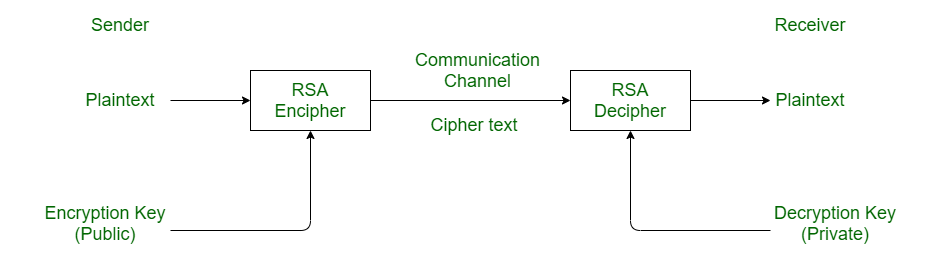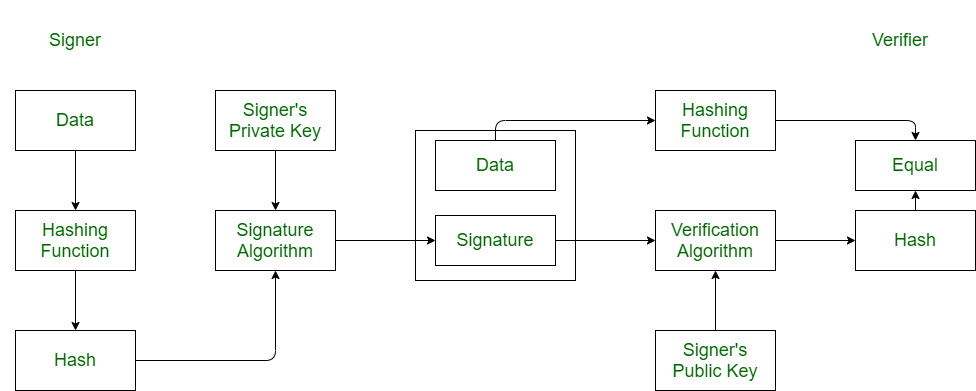# Difference between RSA algorithm and DSA

• Last Updated : 06 Jul, 2022

1. Rivest-Shamir-Adleman (RSA) algorithm :

RSA stands for Rivest-Shamir-Adleman. It is a cryptosystem used for secure data transmission. In RSA algorithm, encryption key is public but decryption key is private. This algorithm is based on mathematical fact that factoring the product of two large prime numbers is not easy. It was developed by Ron Rivest, Adi Shamir and Leonard Adleman in 1977.2. Digital Signature Algorithm (DSA) :

DSA stand for Digital Signature Algorithm. It is used for digital signature and its verification. It is based on mathematical concept of modular exponentiation and discrete logarithm. It was developed by National Institute of Standards and Technology (NIST) in 1991.

It involves four operations:

1. Key Generation
2. Key Distribution
3. Signing
4. Signature VerificationDifference between RSA algorithm and DSA :

My Personal Notes arrow_drop_up